Home/All Courses/CBSE/NCERT/NCERT Solutions For Class 9/NCERT Solution For Class 9 Maths/NCERT Solutions for Class 9 Maths Chapter 13 – Surface Areas and Volumes

## NCERT Solutions for Class 9 Maths Chapter 13 – Surface Areas and Volumes

Ncert Solutions for Class 9 Maths Chapter 13 pdf covers the topic of surface areas and volumes class 9 and the formulas to calculate the areas and volumes of Square, Rectangle, Triangle, Circle. To get more in-depth knowledge on surface areas and volumes class 9 refer to  ncert solutions for class 9 maths chapter 13 pdf download

## Area

The space occupied by a two-dimensional flat surface. It is measure in square units.

Generally, Area can be of two types

(i) Total Surface Area

(ii) Curved Surface Area

## Total Surface Area

Total surface area refers to area including the base(s) and the curved part.

Curved surface area (lateral surface area)

Refers to area of only the curved part excluding it’s base(s).

## Volume

The amount of space, measured in cubic units, that an object or substance occupies.

### Surface area and volume all formulas

The Surface area and volume all formulas are given below:

## Areas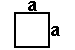square = a2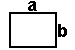rectangle = ab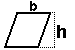parallelogram = bh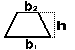trapezoid = h/2 (b1+ b2)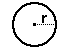circle = pir 2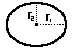ellipse = pir1 r2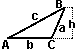• triangle = (1/2) b h
• equilateral triangle = (1/4)(3) a2
• triangle given SAS = (1/2) a b sin C
• triangle given a,b,c = [s(s-a)(s-b)(s-c)] when s = (a+b+c)/2 (Heron’s formula)
• regular polygon = (1/2) n sin(360°/n) S2
when n = # of sides and S = length from center to a corner

## Volumes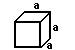cube = a3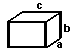rectangular prism = a b c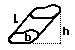irregular prism = bh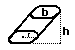cylinder = bh =  r2 h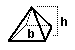pyramid = (1/3) bh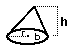Cone = (1/3) bh = 1/3  r2 hsphere = (4/3) r3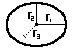ellipsoid = (4/3) pir1 r2 r3

## Surface Areascube = 6 a2prism:
(lateral area) = perimeter(b) L
(total area) = perimeter(b) L + 2bsphere = 4 r2

2018-10-31T11:15:53+00:00 Categories: NCERT Solution For Class 9 Maths||0 Comments
Hello